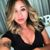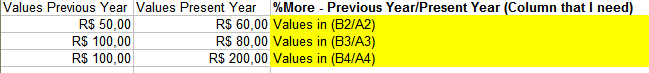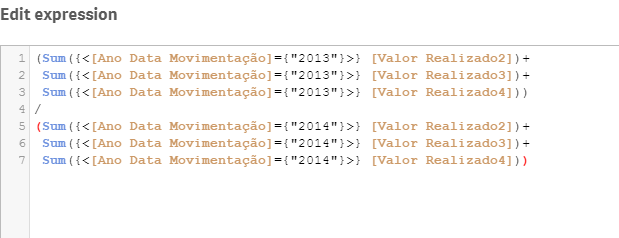# New to Qlik Sense

If you’re new to Qlik Sense, start with this Discussion Board and get up-to-speed quickly.

cancel
Showing results for
Did you mean:Contributor III

## Sum Different columns on a dinamic Table

Hi all,I need to create a column using the following idea:

sum all values on the column 'Valor Realizado' to the year 2013 and divide with the sum all values on the column 'Valor Realizado' to the year 2014

How can I do it? have any Idea.

I tried do use sum(column()) But this is a Dinamic table with so many dimensions.

Hope you can help.

Awaiting.

Thanks!

Amanda Aryel

1 Solution

Accepted SolutionsMaster II

Hi Amanda,

Do you have a master calendar created in your data model. If so it will be quite straight forward to get this calculation done.

use: sum({<CalYear={"=\$(year(today()))"}>} Sum_field) /  sum({<CalYear={"=\$(year(addyears(today(),-1)))"}>} Sum_field)

or if you have flags set for this year and last year

use: sum({<[this Year flag]={1}>} sum_field) / sum({<[Last Year flag]={1}>} sum_field)

To set the flags in the master calendar make use of inYear() function.

Thanks,

Sangram.

7 RepliesSpecialist III

Hi,

You can try something like this:

Sum({<Your_Date_Field={">=01/01/2013<=12/31/2013"}>} Total Your_Field) / Sum({<Your_Date_Field={">=01/01/2014<=12/31/2014"}>} Total Your_Field)

Since I don't know your fields and date formats, the above should give you some pointers.

You can also post some sample data.

Hope this helps.Contributor III
Author

It helps in part,

but using this expression, it show the same value (a total) in each row.

and I need that every row also show the result, just like the column '% Acumulado Total'.

do you have any idea if is possible?Specialist III

Well, that's what Total does. In your original question, you said "sum all values on the column" so Total sums all the values.

Would you happen to have a sample dataset so that we can take a look at it closer?

ThanksContributor III
Author

Yeh, I Got it.

Let me try improve my description.

What I need it is:I need add one more Column '% - Previous Year/ Present Year'

where the calculation is:

'Valor realizado' on the year 2013 divided 'Valor Realizado' on the year 2014.

I don't wanna all values like total does. I need something like the first picture above.

Its the same way that my column '% Acumulado Atual'.

Is it possible?

THANKS

Obs.:

That's why I tried to use the function column().Specialist IIIMaster II

Hi Amanda,

Do you have a master calendar created in your data model. If so it will be quite straight forward to get this calculation done.

use: sum({<CalYear={"=\$(year(today()))"}>} Sum_field) /  sum({<CalYear={"=\$(year(addyears(today(),-1)))"}>} Sum_field)

or if you have flags set for this year and last year

use: sum({<[this Year flag]={1}>} sum_field) / sum({<[Last Year flag]={1}>} sum_field)

To set the flags in the master calendar make use of inYear() function.

Thanks,

Sangram.Contributor III
Author

Thx , it helps me a lot!Tags
Community Browser